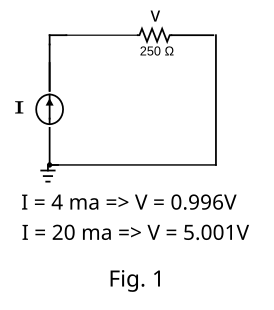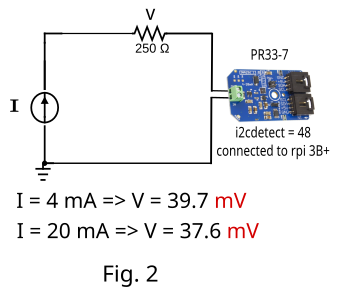# Small current when connecting PR33-7

I have a 4-20 m current generator as show in figure 1.When the current is set to 4 mA , the voltage at the 250 ohm resistor is almost 1V
When the current is set to 20mAm the voltage at th 250 ohm resistor is 5V
This shows that the current generator is working.

Now I have a PR33-7 connected to a Raspberry PI model 3B+ and I get a red ligth on the board. If I use the command i2cdetect -y 1, I get a 48 which is the address of the board.
But If a connect the board to the circuit, like it is shown in Figure 2.When the current is set to 4 mA I get 39.7 mV at the 250 ohm resistor.
When the current is set to 20ma I get 37.6mV at the 250 ohm resistor.

It seems that the impedance of the board is so high that allows a 1.08uA in the circuit.

Can you give some pointers of what can I be doing wrong? Is it possible that the board is defective?